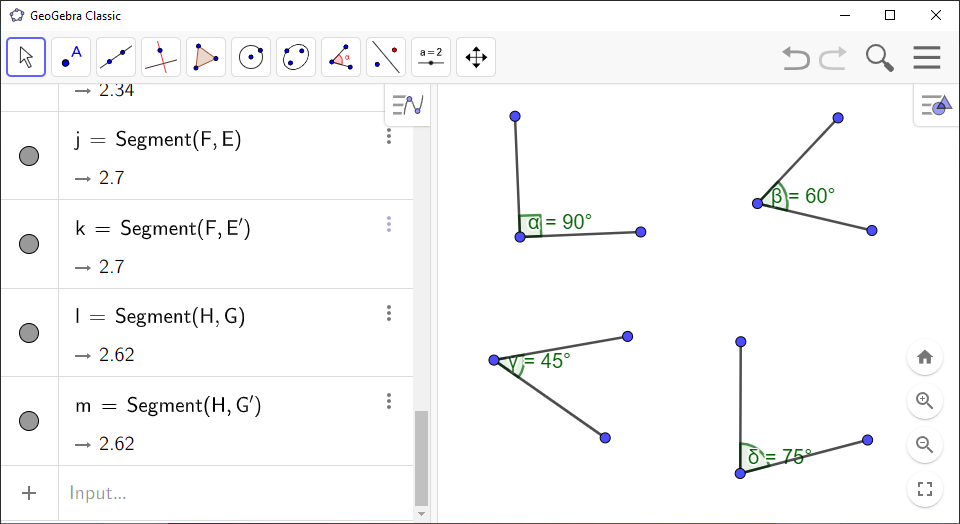Math Topics

# How to Create an Angle in GeoGebra

You can use `GeoGebra` to draw angles. There are two methods you can use: The first method is to specify three points or two lines that define the angle. The second method is to specify the desired size of the angle.

`GeoGebra` Instruction 1

### AngleFormedbyThreePointsorTwoLines

1.
Open `Algebra View` and `Graphics View` under`View` in`Menu`.
2.
If you wish to find the angle between two lines, draw those lines now.
3.
Select the `Angle`tool in `Toolbar`.
4.
Draw the angle in `Graphics View` either by clicking on three points in `Graphics View` or clicking on the two lines from Step 2. Recall that angles are drawn counterclockwise.
5.
The size of the angle will be displayed in `Algebra View`. Notice that the sides of the angle have the same length. If you want to see the sides from the vertex to the endpoints, use the `Segment`tool to draw the segments between the points so that they will be visible. Feel free to experiment drawing angles with different sizes.

`GeoGebra` Instruction 2

### AnglewithGivenSize

1.
Open `Algebra View` and `Graphics View` in under`View` in`Menu`.
2.
Click `Point`in the `Toolbar`.
3.
Click where you want the vertex (top) of the angle to be. A point will be made there.
4.
Then click on a point where you want one of the rays of the angle to go through.
5.
Click `Angle with Given Size`in `Toolbar` by first clicking tool number 8, then tool number 2 in the drop-down list.
6.
Click the point from Step 4 first, then click the point from Step 3.
7.
A box will now appear. Enter how large the angle should be in the text field.

If you want your angle to go clockwise, select the box below the text field.

Click `OK`.

8.
If you want to draw the rays of the angle, then click `Segment`by first clicking tool number 3, then tool number 2. Select the vertex and one of the other points. Repeat once more for the other point.

Example 1

Draw angles with sizes of $\text{}90\text{}\text{°}$, $\text{}60\text{}\text{°}$, $\text{}45\text{}\text{°}$ and $\text{}75\text{}\text{°}$ in `GeoGebra`.

Here, you choose the `Angle with Given Size`tool because it can precisely draw the angles you desire. You therefore follow Item 8 and type in the size of each angle.